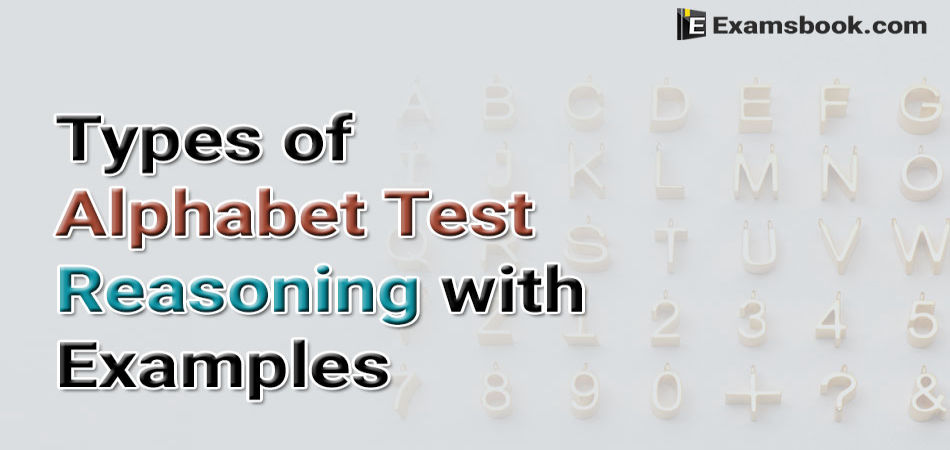• Save

Different Types of Alphabet Test Reasoning with Examples

7 months ago 1.6K ViewsThere are different types of Alphabet test reasoning questions which are asked in the competitive exams. Alphabet test topic has divided into some different types which I am sharing you with examples.

You should know the types of alphabet test to save your time and to obtain a better rank in the competitive exams. After knowing types of alphabet test reasoning, you can start your practice with Alphabet Series Questions and Answers

Types of Alphabet Test Reasoning

Type 1: Alphabetical Order of Words

Alphabetical Order: Arranging words in alphabetical order implies ‘to arrange them in the order as they appear in a dictionary’, i.e., as per the order in which the beginning letters of these words appear in the English alphabet.

Ex.1. If the following five words are arranged in alphabetical order, which word will come in the middle?

(A) Draw

(C) Play

(D) Back

(E) Want

Solution

Arranging the words in alphabetical order, we have: Back, Draw, Play, Read, Want. Clearly, the word ‘Play’ comes in the middle. So, the answer is (c).

Ex.2. Which of the following words will come fourth in the English dictionary?

(A) False

(B) Follow

(C) Faithfully

(D) Fool

(E) Fallible

Solution

The given words can be arranged in the alphabetical order as:

Faithfully, Fallible, False, Follow, Fool

Clearly, ‘Follow’ comes fourth. So, the answer is (b).

Type 2: Letter-Word Problems

Ex.1. How many such pairs of letters are there in the words INSTRUCTION which have as many letters between them in the word as in the English alphabet?

(A) One

(B) Two

(C) Three

(D) Four

(E) None of these

Solution

Clearly, we have:

As depicted above, C and I have five letters between them in the given word as well as in English alphabet; N and T again have five letters between them and each of the pairs ( S and T) and (N and O) have no letter between them.

Thus, there are four such pairs. Hence, the answer is (d).

Ex.2. How many such pairs of letters are there in the word CORPORATE each of which has may letters in the same sequence between them in the word as in the English alphabet?

(A) None

(B) One

(C) Two

(D) Three

(E) More than three

Solution

Three pairs – (P, R), (R, T) and (P, O) have as many letters between them in the word as in the English alphabet. But since the letters must be in the same sequence in the word as in the English alphabet, so the desired pairs are (P, R) and (R, T) only.

Hence the answer is (C)

Type 3: Rule – Detection

Direction (Question 1 to 24): In each of the following questions, find out which of the letter-series follows the given rule.

1. Number of letters skipped in between adjacent letters in the series is two.

(A) MPSVYBE

(B) QSVYZCF

(C) SVZCGJN

(D) ZCGKMPR

Solution

Hence the answer is (a)

2. The group of letters should not contain more than two vowels.

(A) BDEJOLY

(B) JKAPIXU

(C) PRAQEOS

(D) ZILERAM

Solution

B D E J O L Y has only two vowels – E and O.

Hence the answer is (a)

Type 4: Alphabetical Quibble

In this type of question, generally a letter – series is given, be it the English alphabets from. A to Z or a randomized sequence of letters. The candidate is then required to trace the letters satisfying certain given conditions as regards their position in the given sequence or the sequence obtained by performing certain given operation on the given sequence.

Ex.1. Answer the give question based on the following English alphabet:

A B C D E F G H I J K L M N O P Q R S T U V W X Y Z

1. Which letter is fifth to the right of the eighteenth letter from your right?

(A) C

(B) D

(C) E

(D) M

(E) N

Solution

Counting form, the right end of the given alphabet – series, i.e., form Z, the eighteenth letter is I. Counting from I towards the right, the fifth letter is N.

So, the answer is (e).

2. If all the vowels are removed from the alphabet, which letter will be the seventh to the right of the fifth letter from the left?

(A) L

(B) M

(C) N

(D) P

(E) None of these

Solution

Removing all the vowels, i.e., A, E, I, O, U, we obtain the following letters-series:

B C D F G H J K L M N P Q R S T V W X Y Z.

Counting from the left in the above series, i.e., from B, the fifth letter is G. Counting from G towards the right, the seventh letter is P.

So, the answer is (e).